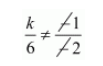# The value of k for which the system of equations has a unique solution, is

Question:

The value of for which the system of equations has a unique solution, is

$k x-y=2$

$6 x-2 y=3$

(a) $=3$

(b) $\neq 3$

(c) $\neq 0$

(d) $=0$

Solution:

The given system of equations are

$k x-y=2$

$6 x-2 y=3$

$\frac{a_{1}}{a_{2}} \neq \frac{b_{1}}{b_{2}}$ for unique solution

Here $a_{1}=k, a_{2}=6, b_{1}=-1, b_{2}=-2$By cross multiply we get

$2 k \neq 6$

$k \neq \frac{6}{2}$

$k \neq 3$

Hence, the correct choice is $b$.